#Basic RulesIn this section, you will learn how so solve inequalities. "Solving'' an inequality means finding all of its solutions. A "solution'' of an inequality is a number which when substituted for the variable makes the inequality a true statement.

Here is an example: Consider the inequality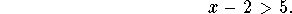When we substitute 8 for x, the inequality becomes 8-2 > 5. Thus, x=8 is a solution of the inequality. On the other hand, substituting -2 for x yields the false statement (-2)-2 > 5. Thus x = -2 is NOT a solution of the inequality. Inequalities usually have many solutions.

As in the case of solving equations, there are certain manipulations of the inequality which do not change the solutions. Here is a list of "permissible'' manipulations:

Rule 1. Adding/subtracting the same number on both sides.

Example: The inequality x-2>5 has the same solutions as the inequality x > 7. (The second inequality was obtained from the first one by adding 2 on both sides.)

Rule 2. Switching sides and changing the orientation of the inequality sign.

Example: The inequality 5-x> 4 has the same solutions as the inequality 4 < 5 - x. (We have switched sides and turned the ``>'' into a ``<'').

Last, but not least, the operation which is at the source of all the trouble with inequalities:

Rule 3a. Multiplying/dividing by the same POSITIVE number on both sides.

Rule 3b. Multiplying/dividing by the same NEGATIVE number on both sides AND changing the orientation of the inequality sign.

Examples: This sounds harmless enough. The inequality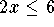has the same solutions as the inequality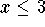. (We divided by +2 on both sides).

The inequality -2x > 4 has the same solutions as the inequality x< -2. (We divided by (-2) on both sides and switched ">'' to "<''.)

But Rule 3 prohibits fancier moves: The inequality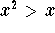DOES NOT have the same solutions as the inequality x > 1. (We were planning on dividing both sides by x, but we can't, because we do not know at this point whether x will be positive or negative!) In fact, it is easy to check that x = -2 solves the first inequality, but does not solve the second inequality.

Only ``easy'' inequalities are solved using these three rules; most inequalities are solved by using different techniques.

Let's solve some inequalities:

#### Example 1:

Consider the inequality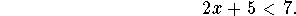The basic strategy for inequalities and equations is the same: isolate x on one side, and put the "other stuff" on the other side. Following this strategy, let's move +5 to the right side. We accomplish this by subtracting 5 on both sides (Rule 1) to obtain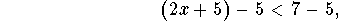after simplification we obtain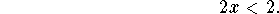Once we divide by +2 on both sides (Rule 3a), we have succeeded in isolating x on the left: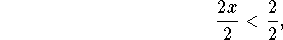or simplified,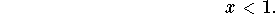All real numbers less than 1 solve the inequality. We say that the "set of solutions'' of the inequality consists of all real numbers less than 1. In interval notation, the set of solutions is the interval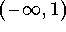.

#### Example 2:

Find all solutions of the inequality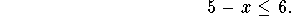Let's start by moving the ``5'' to the right side by subtracting 5 on both sides (Rule 1):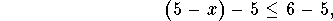or simplified,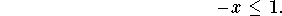How do we get rid of the ``-'' sign in front of x? Just multiply by (-1) on both sides (Rule 3b), changing "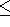" to "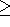" along the way: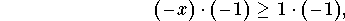or simplified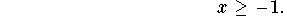All real numbers greater than or equal to -1 satisfy the inequality. The set of solutions of the inequality is the interval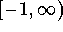.

#### Example 3:

Solve the inequality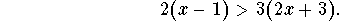Let us simplify first: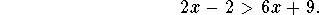There is more than one route to proceed; let's take this one: subtract 2x on both sides (Rule 1).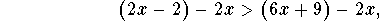and simplify: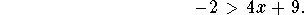Next, subtract 9 on both sides (Rule 1):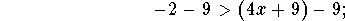simplify to obtain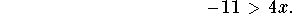Then, divide by 4 (Rule 3a):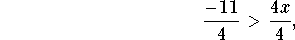and simplify again: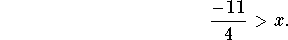It looks nicer, if we switch sides (Rule 2).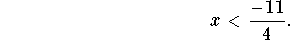In interval notation, the set of solutions looks like this: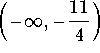.

#### Exercise 1:

Find all solutions of the inequality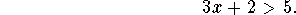#### Exercise 2:

Solve the inequality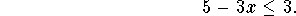#### Exercise 3:

Solve the inequality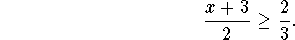#### Exercise 4:

Find all solutions of the inequality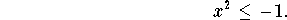[Back] [Next]
[Algebra] [Trigonometry] [Complex Variables]
[Calculus] [Differential Equations] [Matrix Algebra]S.O.S MATHematics home page

Do you need more help? Please post your question on our S.O.S. Mathematics CyberBoard.